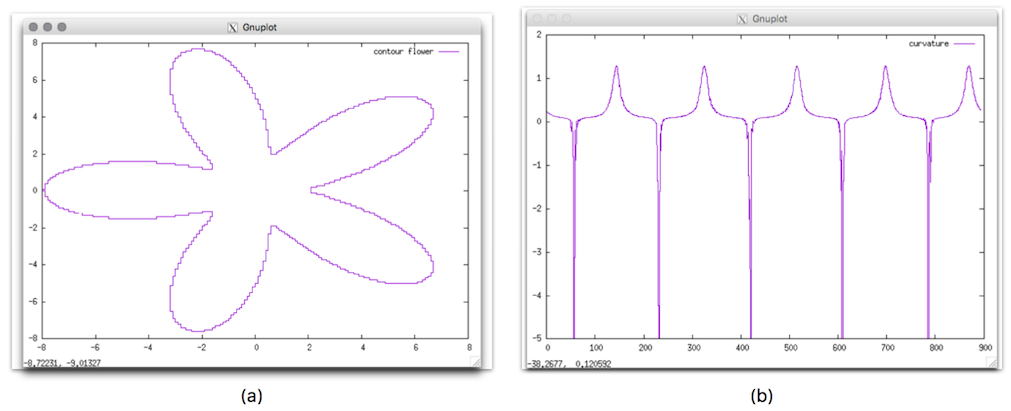DGtalTools  1.3.beta
contourGenerator

Generates multigrid contours of 2d digital shapes using DGtal library.

The associated geometric information like contour, curvature can also be displayed.

Usage: contourGenerator –shape <shapeName> [requiredParam] [otherOptions]

Allowed options are:

-h,--help Print this help message and exit
-l,--list List all available shapes
-s,--shape TEXT Shape name
-A,--axis1 FLOAT Half big axis of the shape (ellipse)
-a,--axis2 FLOAT Half small axis of the shape (ellipse)
-r,--smallradius FLOAT=5 Small radius of the shape (default 5)
-v,--varsmallradius FLOAT=5 Variable small radius of the shape (default 5)
-k UINT=3 Number of branches or corners the shape (default 3)
--phi FLOAT=0 Phase of the shape (in radian, default 0.0)
-w,--width FLOAT=10 Width of the shape (default 10.0)
-p,--power FLOAT=2 Power of the metric (default 2.0)
-x,--center_x FLOAT=0 x-coordinate of the shape center (default 0.0)
-y,--center_y FLOAT=0 y-coordinate of the shape center (default 0.0)
-g,--gridstep FLOAT=1 Gridstep for the digitization (default 1.0)
-f,--format TEXT=pts Output format:
List of pointel coordinates {pts}
Freeman chaincode Vector {fc} (default pts)
-o,--outputGeometry TEXT Base name of the file containing the shape geometry (points, tangents, curvature)

You can also list all possible shapes:

contourGenerator --list
2D Shapes:
ball Ball for the Euclidean metric.
Required parameter(s): --radius [-R]
square square (no signature).
Required parameter(s): --width [-w]
lpball Ball for the l_power metric (no signature).
Required parameter(s): --radius [-R], --power [-p]
flower Flower with k petals with radius ranging from R+/-v.
Required parameter(s): --radius [-R], --varsmallradius [-v], --k [-k], --phi
ngon Regular k-gon.
Required parameter(s): --radius [-R], --k [-k], --phi
accflower Accelerated Flower with k petals.
Required parameter(s): --radius [-R], --varsmallradius [-v], --k [-k], --phi
ellipse Ellipse.
Required parameter(s): --axis1 [-A], --axis2 [-a], --phi

Example:

# generate a flower contour with 5 petals of maximal radius 5 and small radius 3 with grid size = 0.1:
./shapeGenerator/contourGenerator -s flower -R 5 -v 3 -k 5 --outputGeometry result -g 0.1
# Display the results using gnuplot:
\$gnuplot
[gnuplot> plot [][-5:2]'res.geom' using 1:6 w l t "curvature"
[gnuplot> plot 'res.geom' using 2:3 w l t "contour flower"

You should obtain such a visualization:resulting visualisation of generated contour (a) and curvature (b).